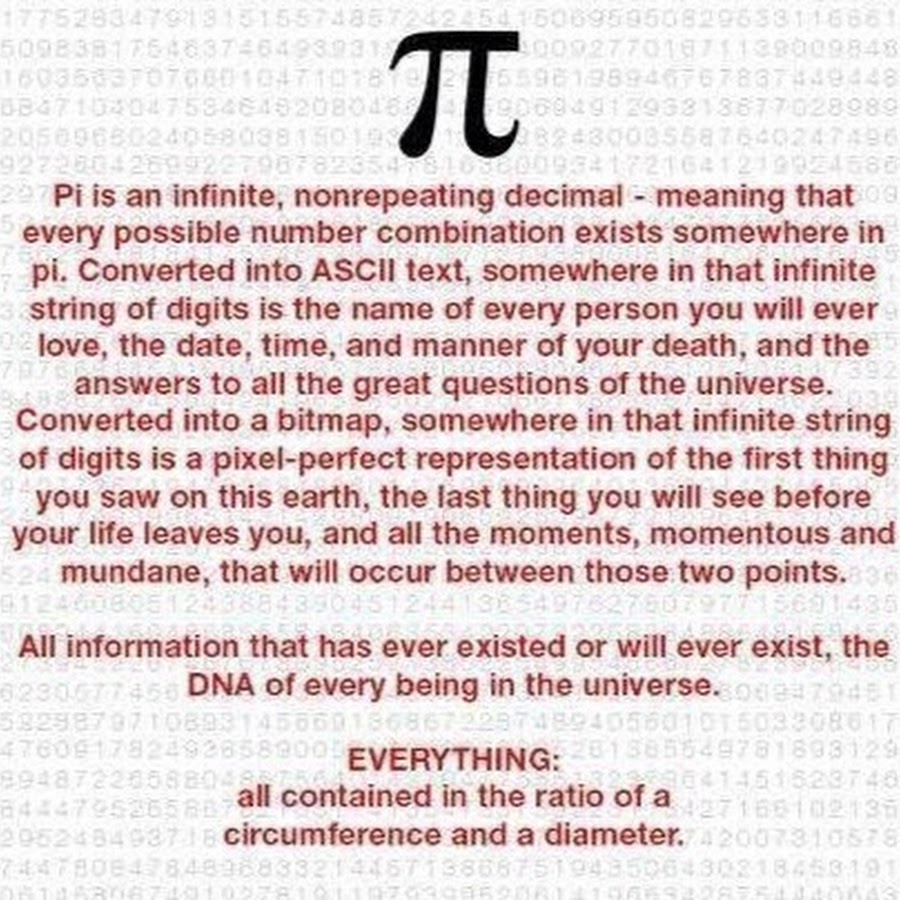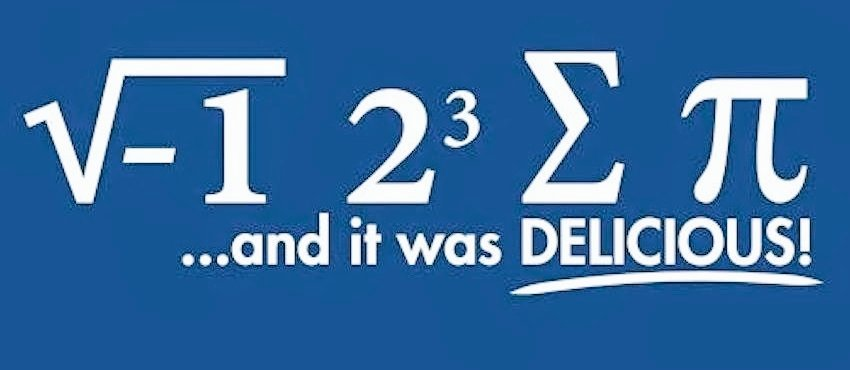# World Pi Day!

Pi is an irrational number having a value of 3.14159 and is a symbolic representation of the ratio of a circle’s circumference to its diameter. This number was devised by the Greek mathematician and polymath Archimedes. There is an annual celebration of this mathematical constant on March 14th (3/14) which is now called as “Pi Day”.

Here are some fun Pi facts to enjoy on this Pi Day:

1. Pi is the 16th letter of the Greek alphabet
2. π has been calculated to over a trillion digits beyond the decimal point and continues infinitely.
3. If you want to see the value of π till one million digits click here
4. Albert Einstein was also born on Pi Day. Just coincidence?
5. The value 3.14, when seen in a mirror, reads as PIE.
6. Pi can save the human race from being destroyed by robots. How? Simply ask the robot to compute the value of pi to the last digit. Ask Spock from Star Trek if you don’t believe me!
7. The infinite string of numbers in π is also believed to contain the secrets of the universe and the answers to all unanswered questions. Some food for thought###### Original source unknown
1. Pi has a movie based on it that portrayed the mental state of an obsessive-compulsive mathematician looking for answers in its vast string of numbers. This film was direct by Darren Aronofsky and released in 1998.

Here’s a riddle to solve while you enjoy some delicious pie! 🙂###### Original source unknown

1 Comment
1.aetzbar says

Archimedes determined that a single pi number (3.14) belongs to all circles.
All the mathematicians who came after Archimedes agreed with him.
Universities teach the idea of a single pi number.
The mathematicians even invented proof of the idea of a single pi number.
But mathematicians are wrong, and each circle has a unique pi number.
This is pi revolution , that science has been waiting for 2000 years

X

• 2000+ blog articles
• 50+ Webinars
• 10+ Expert podcasts
• 50+ Infographics
• Q&A Forum
• 10+ eBooks
• 10+ Checklists
• Research Guides
[contact-form-7 id="40123" title="Global popup two"]

Which is the best strategy to support research integrity according to you?